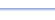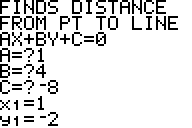Program: PT2LINE

PT2LINE

• Finds the distance between a point and a line.

Useful for:

• SAT
• ACT
• Algebra 1
• Algebra 2
• Geometry
• Precalculus
• Calculus
• College Algebra

See full list of programs

Example

Q: Find the distance from the point (1, -2) and the line x + 4y = 8.

Solution:

First, rewrite the equation of the line in the form
Ax + By + C = 0:

1x + 4y - 8 = 0

Run PT2LINE.  How?

Enter the coefficients
for A, B, and C.

Enter the x and y
values from the
ordered pair.

The program reports:

Interpretation:  The distance is about 3.6.